# How to Simplify √1176

About 83% of numbers whose square root can be simplified are divisible by 4 or by 9 or by both. It’s easy to see rather quickly if a number is divisible by either of them. I want to find square roots by doing as few divisions as possible. It is easier to divide a number by 4 than it is to divide it by 2 twice. Likewise, it is easier to divide a number by 9 than it is to divide it by 3 twice.

1176 is divisible by 4 because the number formed from its last two digits, 76, is divisible by 4. So let’s do that division:
1176÷4=294.

Can we divide 294 by 4? We can quickly see that 94 is not divisible by 4, so 294 is not divisible by 4. Let’s check to see if it is divisible by nine: 2 + 9 + 4 = 15, which is not divisible by 9 but is by 3. That means that 294 is not divisible by 9, but it is divisible by 3.

Since 294 is an even number that is divisible by 3, I will save time and make fewer mistakes if I just divide it by 6 instead of by 2 and then by 3.
294÷6 = 49

49 is a perfect square so I know I’ve done enough dividing for this particular number. I’ve made a gif to illustrate the steps I’ve just explained:make science GIFs like this at MakeaGif

By doing just a little bit of arithmetic in my head, I can also tell by looking at that cake that the prime factorization of 1176 is 2³ × 3 × 7².

Some people still prefer to use factor trees to find square roots. Here are a few of its MANY possible trees.Here’s more about the number 1176:

• 1176 is a composite number.
• Prime factorization: 1176 = 2 × 2 × 2 × 3 × 7 × 7, which can be written 1176 = 2³ × 3 × 7²
• The exponents in the prime factorization are 3, 1 and 2. Adding one to each and multiplying we get (3 + 1)(1 + 1)(2 + 1) = 4 × 2 × 3 = 24. Therefore 1176 has exactly 24 factors.
• Factors of 1176: 1, 2, 3, 4, 6, 7, 8, 12, 14, 21, 24, 28, 42, 49, 56, 84, 98, 147, 168, 196, 294, 392, 588, 1176
• Factor pairs: 1176 = 1 × 1176, 2 × 588, 3 × 392, 4 × 294, 6 × 196, 7 × 168, 8 × 147, 12 × 98, 14 × 84, 21 × 56, 24 × 49 or 28 × 42
• Taking the factor pair with the largest square number factor, we get √1176 = (√196)(√6) = 14√6 ≈ 34.292861176 is the sum of the first 48 numbers so we say it the 48th triangular number. We know it is the sum of the first 48 numbers because (48 × 49)/2 = 1176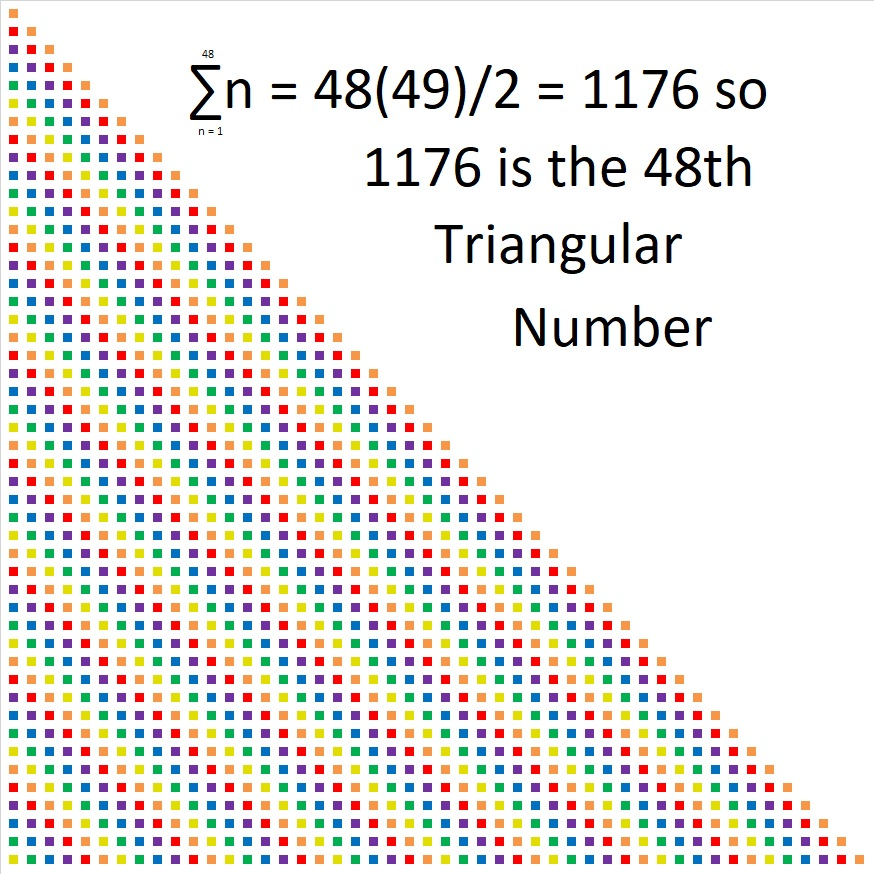1176 looks interesting in a few other bases:
It’s 3300 in BASE 7 because 3(7³ + 7²) = 3(392) = 1176,
6C6 in BASE 13 (C is 12 base 10) because 6(13²) + 12(13) + 6(1) = 1176,
600 in BASE 14 because 6(14²) = 1176,
1M1 in BASE 25 (M is 22 base 10) because 25² + 22(25) + 1 = 1176
and it looks like one of its factors, 196, in BASE 30
because 1(30²) + 9(30) + 6(1) = 1176

What’s special about √1024? Is it because it and several counting numbers after it have square roots that can be simplified?Perhaps.

Maybe it is interesting just because √1024 = 32, a whole number. The 5th root of 1024 = 4 and the 10th root of 1024 = 2, both whole numbers as well.

Those equations are true because 32² = 1024, 4⁵ = 1024, and 2¹⁰ = 1024.

Or perhaps 1024 is special because it is the smallest number that is a 10th power. (It is 2¹⁰.) The square root of a perfect 10th power is always a perfect 5th power. (32 = 2⁵ and is the smallest number that is a 5th power.)

1024 is also the smallest number with exactly 11 factors.

It is the smallest number whose factor tree has at least 10 leaves that are prime numbers. (They are the red leaves on the factor tree shown below.) It is possible to draw several other factor trees for 1024, but they will all have the number 2 appearing ten times.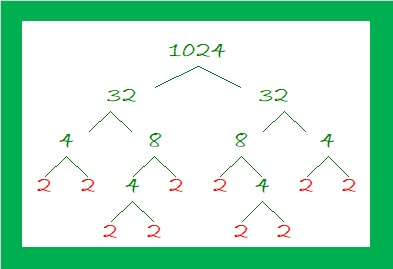What’s more, I noticed something about 1024 and some other multiples of 256: Where do multiples of 256 fall on the list of square roots that can be simplified?

• 256 × 1 = 256 and 256 is the 100th number on this list of numbers whose square roots can be simplified.• 256 × 2 = 512. When we add the next 100 square roots that can be simplified, 512 is the 199th number on the list.• Here are the third 100 square roots that can be simplified: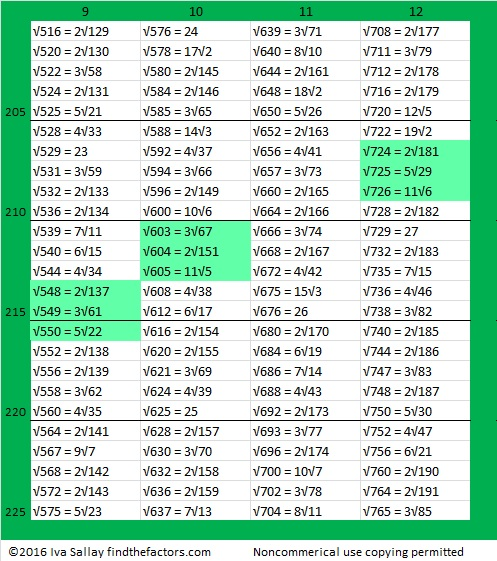• 256 × 3 = 768 didn’t quite make that list because it is the 301st number. Indeed, it is the first number on this list of the fourth 100 numbers whose square roots can be simplified.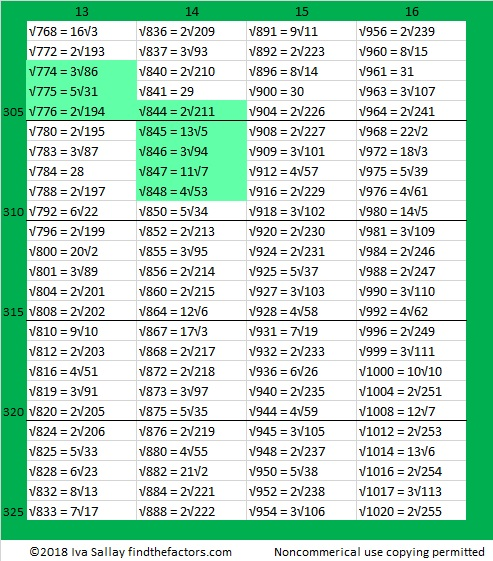• 256 × 4 = 1024. That will be the first number on the 5th 100 square roots list!

It is interesting that those multiples of 256 have the 100th, the 199th, the 301st, and the 401st positions on the list. That is so close to the 100th, 200th, 300th, and 400th positions.

In case you couldn’t figure it out, the highlighted square roots are three or more consecutive numbers that appear on the list.

1024 is interesting for many other reasons. Here are a few of them:

(4-2)¹⁰ = 1024, making 1024 the 16th Friedman number.

I like to remember that 2¹⁰ = 1024, which is just a little bit more than a thousand. Likewise 2²⁰ = 1,048,576 which is about a million. 2³⁰ is about a billion, and 2⁴⁰ is about a trillion.

*******
As stated in the comments, Paula Beardell Krieg shared a related post with me. It takes exactly 1024 Legos to build this fabulous pyramidal fractal:

1024 has so many factors that are divisible by 4 that it is a leg in NINE Pythagorean triples:
768-1024-1280 which is (3-4-5) times 256
1024-1920-2176 which is (8-15-17) times 128
1024-4032-4160 which is (16-63-65) times 64
1024-8160-8224 which is (32-255-257) times 32
1024-16368-16400 which is 16 times (64-1023-1025)
1024-32760-32776 which is 8 times (128-4095-4097)
1024-65532-65540 which is 4 times (256-16383-16385)
1024-131070-131074 which is 2 times (512-65535-65537),
and primitive 1024-262143-262145

Some of those triples can also be found because 1024 is the difference of two squares four different ways:
257² – 255² = 1024
130² – 126² = 1024
68² – 60² = 1024
40² – 24² = 1024
To find out which difference of two squares go with which triples, add the squares instead of subtracting and you’ll get the hypotenuse of the triple.
******

1024 looks interesting in some other bases:
It’s 1000000000 in BASE 2,
100000 in BASE 4,
2000 in BASE 8,
1357 in BASE 9,
484 in BASE 15,
400 in BASE 16,
169 in BASE 29,
144 in BASE 30,
121 in BASE 31, and
100 in BASE 32

• 1024 is a composite number.
• Prime factorization: 1024 = 2 × 2 × 2 × 2 × 2 × 2 × 2 × 2 × 2 × 2, which can be written 1024 = 2¹⁰
• The exponent in the prime factorization is 10. Adding one we get (10 + 1) = 11. Therefore 1024 has exactly 11 factors.
• Factors of 1024: 1, 2, 4, 8, 16, 32, 64, 128, 256, 512, 1024
• Factor pairs: 1024 = 1 × 1024, 2 × 512, 4 × 256, 8 × 128, 16 × 64, or 32 × 32,
• 1024 is a perfect square. √1024 = 32. It is also a perfect 5th power, and a perfect 10th power.# 700 Pick Your Pony! Who will win this Amount of Factors Horse Race?

• 700 is a composite number.
• Prime factorization: 700 = 2 x 2 x 5 x 5 x 7, which can be written 700 = (2^2) x (5^2) x 7
• The exponents in the prime factorization are 2, 2 and 1. Adding one to each and multiplying we get (2 + 1)(2 + 1)(1 + 1) = 3 x 3 x 2 = 18. Therefore 700 has exactly 18 factors.
• Factors of 700: 1, 2, 4, 5, 7, 10, 14, 20, 25, 28, 35, 50, 70, 100, 140, 175, 350, 700
• Factor pairs: 700 = 1 x 700, 2 x 350, 4 x 175, 5 x 140, 7 x 100, 10 x 70, 14 x 50, 20 x 35 or 25 x 28
• Taking the factor pair with the largest square number factor, we get √700 = (√100)(√7) = 10√7 ≈ 26.457513.Because this is my 700th post, I think I’ll have another horse race. Some numbers from 601 to 700 have exactly 2 factors, 4 factors, and so forth up to 24 factors. (Only perfect squares can have an odd number of factors.)

Which number from 1 to 24 will win this amount of factors horse race? Which number will come in second place, or third place? Cheering for more than one pony will make the race even more interesting.

Here we see that the numbers 2, 6, & 8 are the first ones out of the gate. Click on the graphic to see the rest of this very thrilling horse race:Every hundred posts I also like to focus on the percentage of numbers whose square roots can be simplified.

700 is divisible by 100 so its square root can easily be simplified: √700 = 10√7.

273 of the first 700 numbers have reducible square roots. That’s exactly 39%.

The rest of the numbers, 427, which is 41% of the first 700 numbers, do not have reducible square roots.

Here’s a table breaking down the amount of factors in each group of one hundred integers and the number of reducible square roots.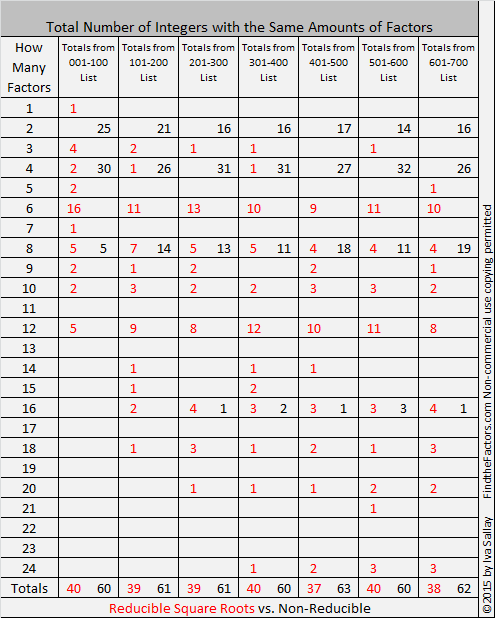Here are some facts about the number 700.

700 is a palindrome in several bases:

• 4A4 BASE 12; note A is equivalent to 1o in base 10, and 4(144) + 10(12) + 4(1) = 700
• PP BASE 27; note P is equivalent to 25 in base 10, and 25(27) + 25(1) = 700
• KK Base 34; note K is equivalent to 20 in base 10, and 20(34) + 20(1) = 700

700 is the sum of four consecutive prime numbers: 167 + 173 + 179 + 181.

Here is a beautiful painting of a horse race that I saw on twitter:

# Simplifying √588 and Level 3

88 is divisible by 4 so 588 is also divisible by 4, and that means √588 can be reduced.

About 83% of the numbers that have reducible square roots are divisible by 4 and/or by 9, and it is so easy to tell if even a very long number is divisible by either of those numbers. It is also easier to divide a number by 4 or 9 than it is to divide by their square roots twice.

When I reduce a square root, I like to make a little cake and start by dividing by 100, 4, or 9 if any of those numbers are its factors. Here are the steps I used to make a cake for 588 with as many perfect squares on the outside of the cake as possible.

1. 588 ÷ 4 = 147
2. 147 is not divisible by 4 again, but 5 + 8 + 8 = 21 so 147 is divisible by 3, but not by 9.
3. 147 ÷ 3 = 49 which is a perfect square, so I stop dividing and simply take the square roots of everything on the outside of the cake and multiply them together.

This is what my cake looks like:And now for today’s Level 3 puzzle:Print the puzzles or type the solution on this excel file: 10 Factors 2015-08-17

—————————————————————————————————

• 588 is a composite number.
• Prime factorization: 588 = 2 x 2 x 3 x 7 x 7, which can be written 588 = (2^2) x 3 x (7^2)
• The exponents in the prime factorization are 2, 1 and 2. Adding one to each and multiplying we get (2 + 1)(1 + 1)(2 + 1) = 3 x 2 x 3 = 18. Therefore 588 has exactly 18 factors.
• Factors of 588: 1, 2, 3, 4, 6, 7, 12, 14, 21, 28, 42, 49, 84, 98, 147, 196, 294, 588
• Factor pairs: 588 = 1 x 588, 2 x 294, 3 x 196, 4 x 147, 6 x 98, 7 x 84, 12 x 49, 14 x 42 or 21 x 28
• Taking the factor pair with the largest square number factor, we get √588 = (√196)(√3) = 14√3 ≈ 24.248711—————————————————————————————————

A Logical Approach to solve a FIND THE FACTORS puzzle: Find the column or row with two clues and find their common factor. Write the corresponding factors in the factor column (1st column) and factor row (top row).  Because this is a level three puzzle, you have now written a factor at the top of the factor column. Continue to work from the top of the factor column to the bottom, finding factors and filling in the factor column and the factor row one cell at a time as you go.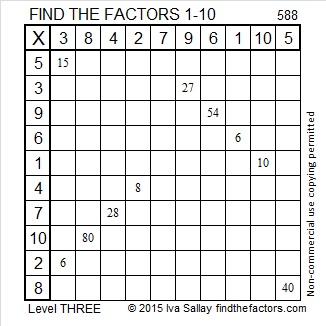# Square Roots Up to √513 That Can Be Simplified

5 + 1 + 3 = 9 so 513 can be evenly divided by 9, and thus its square root can be simplified.

513 is the 200th counting number whose square root can be reduced. 200/513 ≈ .38986, which means, so far, 38.97% of the counting numbers have reducible square roots.

Here are the first 100 reducible square roots followed by the second hundred:I highlighted the ones that are part of three or more consecutive reducible square roots.• 513 is a composite number.
• Prime factorization: 513 = 3 x 3 x 3 x 19, which can be written 513 = (3^3) x 19
• The exponents in the prime factorization are 3 and 1. Adding one to each and multiplying we get (3 + 1)(1 + 1) = 4 x 2 = 8. Therefore 513 has exactly 8 factors.
• Factors of 513: 1, 3, 9, 19, 27, 57, 171, 513
• Factor pairs: 513 = 1 x 513, 3 x 171, 9 x 57, or 19 x 27
• Taking the factor pair with the largest square number factor, we get √513 = (√9)(√57) = 3√57 ≈ 22.6495033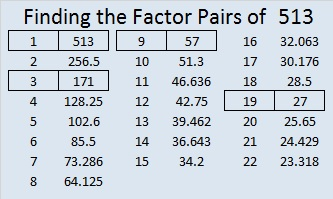# How to Simplify √450

What’s easier – dividing a number by 2 twice or dividing by 4 once? Most people would agree that dividing by a single digit number like 9 one time is easier than dividing by 3 two times. It also cuts the chance of making a mistake in half.

To simplify square roots, I’ve modified the cake method to look for some specific SQUARE factors rather than beginning with ALL of its prime factors.

Divisibility tricks let me know which square factors to try. I always divide out easy and very common perfect squares 100, 4, 9, and 25 first. (About 82% of reducible square roots are divisible by 4 and/or 9.) Once any of those that apply have been divided out, I look to see if 6 or 10 can be divided out because its easier to divide by 6 or 10 once than to divide by 2 and then by 3 or 5. Only after I have divided out those very easy divisors will I look to divide out any remaining prime factors 2, 3, 5, 7, 11, and so forth. Let me demonstrate this method to simplify √450.

• 450 doesn’t end in 00, so it’s not divisible by 100.
• The last two digits, 50, are not divisible by 4, so 450 is not divisible by 4.
• 4 + 5 + 0 = 9, so 450 is divisible by 9. Therefore, I do a simple division problem, 450 ÷ 9 = 50, leaving room on the page to do any other needed division problems above it.
• The previous quotient, 50, is not divisible by 9, but any number ending in 25, 50, or 75 is divisible by 25, so I divide it by 25 and get 2. Now I am finished dividing.
• The numbers on the outside of the cake are 9, 25, and 2. I take the square root of each of those numbers and get 3, 5, and √2. The product of those square roots is 15√2. Thus √450 = 15√2.If the number had been something larger like 925, I could multiply 925 by .04 to get 37. Multiplying by .04 is the same as dividing by 25.

Most people have been taught to use a factor tree to find square roots. This is probably 450’s most common factor tree:I like how much more compact and clear this modified cake method is instead. It may take some practice to get used to it, but I will show more examples of it in the future to make it more familiar.

The following puzzle doesn’t have anything to do with the number 450 except it’s the number I gave it to distinguish it from every other puzzle I make. I put the factors of 450 immediately after the puzzle to separate the puzzle from its solution that I add to the post the next day.Print the puzzles or type the factors on this excel file:  12 Factors 2015-04-06

——————————————————————————————————————-

• 450 is a composite number.
• Prime factorization: 450 = 2 x 3 x 3 x 5 x 5, which can be written 450 = 2 x (3^2) x (5^2)
• The exponents in the prime factorization are 1, 2 and 2. Adding one to each and multiplying we get (1 + 1)(2 + 1)(2 + 1) = 2 x 3 x 3 = 18. Therefore 450 has exactly 18 factors.
• Factors of 450: 1, 2, 3, 5, 6, 9, 10, 15, 18, 25, 30, 45, 50, 75, 90, 150, 225, 450
• Factor pairs: 450 = 1 x 450, 2 x 225, 3 x 150, 5 x 90, 6 x 75, 9 x 50, 10 x 45, 15 x 30 or 18 x 25
• Taking the factor pair with the largest square number factor, we get √450 = (√225)(√2) = 15√2 ≈ 21.2132——————————————————————————————————————-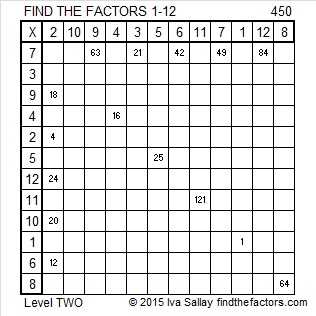# Square Roots up to √352 That Can Be Simplified

• 352 is even and therefore a composite number.
• Prime factorization: 352 = 2 x 2 x 2 x 2 x 2 x 11, which can be written 352 = (2^5) x 11
• The exponents in the prime factorization are 5 and 1. Adding one to each and multiplying we get (5 + 1)(1 + 1) = 6 x 2 = 12. Therefore 352 has exactly 12 factors.
• Factors of 352: 1, 2, 4, 8, 11, 16, 22, 32, 44, 88, 176, 352
• Factor pairs: 352 = 1 x 352, 2 x 176, 4 x 88, 8 x 44, 11 x 32, or 16 x 22
• Taking the factor pair with the largest square number factor, we get √352 = (√16)(√22) = 4√22 ≈ 18.762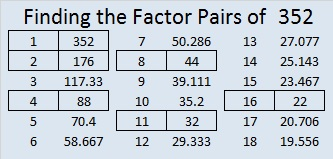Mathematics is full of interesting patterns. Let’s explore some patterns in reducible square roots.

Here are the first ten numbers that have reducible square roots. Notice that five of the numbers in this list, 1, 4, 9, 16, and 25 are perfect squares:40% of the numbers up to 5 have reducible square roots. The same thing is true for 40% of the numbers up to 10, 40% of the numbers up to 20, and 40% of the numbers up to 25.

Here are the second ten numbers with reducible square roots. The last number,50, is double the previous last number, 25. Again 40% of the numbers up to 50 have reducible square roots, and only two of these numbers are perfect squares. Notice that the last three numbers under the radical sign in this set are consecutive numbers.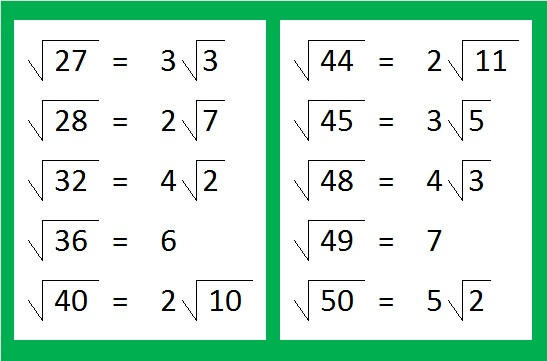Would you like to make any predictions for the third set of ten numbers with reducible square roots?

If you predicted that this set of numbers would end with 75, you were almost right! 29/75 or 38.67% of the numbers up to 75 have reducible square roots, and 30/76 or 39.47% of the numbers up to 76 do. Both of these values are very close to 40%, but not quite there. Notice this time only one number is a perfect square.Would you like to make a prediction for what will happen with the fourth set of ten numbers with reducible square roots?

Surprise! We’re back to 40% of the numbers up to 100 have reducible square roots. Those consecutive numbers at the end of the set really helped raise the percentage right at the last minute. This set of numbers has two perfect squares.What do you think will happen if we look at all the reducible square roots up to 350? Multiples of any perfect square will always have reducible square roots. Since we have more perfect squares, do you think more will be reducible?

Because the square roots of 351 and 352 are also reducible, let’s include them in this chart. Each column has 20 reducible square roots in it, and they are grouped into fives for easier counting. I’ve highlighted sets of three or four consecutive numbers. In all, we now have charts showing the first 140 reducible square roots. This last set has eight perfect squares. Let’s look at the percentages at the end of some of those sets of consecutive numbers: 50/126 or 39.68% of the first 126 numbers have reducible square roots. 96/245 or 39.18% of the first 245 numbers have reducible square roots. Finally, 140/352 or 39.77% of the numbers up to 352 have reducible square roots. That one is so close to 40%!

No matter how big a table we make, the percentage of reducible square roots will be very close to 40%. It will not get significantly higher because, believe it or not, most numbers are either prime numbers or the product of two or more DIFFERENT primes and thus have square roots that are NOT reducible.

# Integers (up to 300) with the Same Number of Factors

• 300 is a composite number.
• Prime factorization: 300 = 2 x 2 x 3 x 5 x 5, which can be written 300 = (2^2) x 3 x (5^2)
• The exponents in the prime factorization are 2, 1 and 2. Adding one to each and multiplying we get (2 + 1)(1 + 1)(2 + 1) = 3 x 2 x 3 = 18. Therefore 300 has exactly 18 factors.
• Factors of 300: 1, 2, 3, 4, 5, 6, 10, 12, 15, 20, 25, 30, 50, 60, 75, 100, 150, 300
• Factor pairs: 300 = 1 x 300, 2 x 150, 3 x 100, 4 x 75, 5 x 60, 6 x 50, 10 x 30, 12 x 25 or 15 x 20
• Taking the factor pair with the largest square number factor, we get √300 = (√3)(√100) = 10√3 ≈ 17.321I made a couple of graphics a few weeks ago anticipating my 300th post.Observations:

• The only number with exactly one factor is one.
• The number of factors of all the black integers on the chart are powers of 2. These integers have irreducible square roots.
• Consecutive integers with the same number of factors can only occur if the number of factors is NOT a prime number.
• 90/300 or 30% of the first 300 integers have 4 factors. (Largest group)
• 62/300 or 20.6666% of the first 300 integers are prime numbers and therefore have 2 factors. (2nd largest group)
• 49/300 or 16.3333% of the first 300 integers have 8 factors. (3rd largest group)
• 40/300 or 13.3333% of the first 300 integers have 6 factors. (4th largest group) All integers with 6 factors have reducible square roots.
• 22/300 or 7.3333% of the first 300 integers have 12 factors. (5th largest group)
• All but 37/300 or 12.3333% of the first 300 integers are in one of those 5 groups.
• 118/300 or 39.3333% of the first 300 integers have square roots that can be simplified.

Warning: the next chart and observations could make your brain hurt:• How many numbers have exactly 2 factors? Euclid proved that there is an infinite number of prime numbers which means there is an infinite number of integers with exactly 2 factors.
• How many integers have exactly 19 factors? Even though the smallest integer with exactly 19 factors is 262,144, there is still an infinite number of integers with exactly that many factors. The integers in that list are each prime number raised to the 18th power. Since there is an infinite number of prime numbers, there is an infinite number of integers with exactly 19 factors.
• {2⁹⁹⁶, 3⁹⁹⁶, 5⁹⁹⁶, . . . } is the infinite list of integers with exactly 997 factors. Likewise {2ᵖ⁻¹, 3ᵖ⁻¹, 5ᵖ⁻¹, . . . } where p is a prime number is the infinite list of integers with exactly p factors.
• If the number of factors is c, a composite number, then it could be said that there is more than an infinite number of integers with that many factors because the infinite list of integers will include {2ᶜ⁻¹, 3ᶜ⁻¹, 5ᶜ⁻¹, . . . } as well as many other integers.

# 281 and More Consecutive Square Roots

• 281 is a prime number.
• Prime factorization: 281 is prime.
• The exponent of prime number 281 is 1. Adding 1 to that exponent we get (1 + 1) = 2. Therefore 281 has exactly 2 factors.
• Factors of 281: 1, 281
• Factor pairs: 281 = 1 x 281
• 281 has no square factors that allow its square root to be simplified. √281 ≈ 16.763How do we know that 281 is a prime number? If 281 were not a prime number, then it would be divisible by at least one prime number less than or equal to √281 ≈ 16.763. Since 281 cannot be divided evenly by 2, 3, 5, 7, 11, or 13, we know that 281 is a prime number.

So far I have posted about one set of four and two sets of five consecutive reducible square roots. Sets of three consecutive reducible square roots are fairly common so I’ve ignored most of them. These consecutive square roots couldn’t be ignored:The final square root features today’s prime number 281. Here are the prime factorizations and number of factors of each of these numbers:Six is a popular number when counting the number of factors.

# 269 and Five More Consecutive Square Roots

• 269 is a prime number.
• Prime factorization: 269 is prime.
• The exponent of prime number 269 is 1. Adding 1 to that exponent we get (1 + 1) = 2. Therefore 269 has exactly 2 factors.
• Factors of 269: 1, 269
• Factor pairs: 269 = 1 x 269
• 269 has no square factors that allow its square root to be simplified. √269 ≈ 16.401How do we know that 269 is a prime number? If 269 were not a prime number, then it would be divisible by at least one prime number less than or equal to √269 ≈ 16.401. Since 269 cannot be divided evenly by 2, 3, 5, 7, 11, or 13, we know that 269 is a prime number.

As I have previously written, 844, 845, 846, 847, and 848 are the smallest FIVE consecutive numbers whose square roots can be simplified. Here are the second smallest FIVE with the same property.The first number in the second set, 1680, equals 2 x 840 which is very close to the first number in the first set. Will strings of five consecutive numbers with reducible square roots occur about once every 850 numbers?

We can find the number of factors for these numbers by examining their prime factorizations.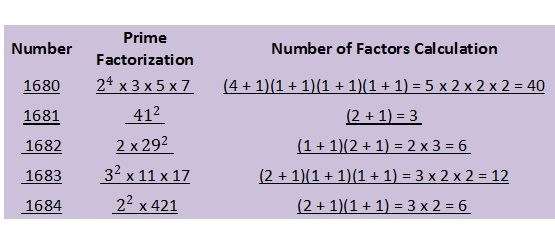The number of factors for each of the integers in this second set ranges from 3 to 40. Only two of the integers have the same number of factors. Finding another string of four or more numbers that have reducible square roots as well as the same number of factors may be difficult.